RRB JE ME (CBT I) Mock Test- 1

# RRB JE ME (CBT I) Mock Test- 1

Test Description

## 100 Questions MCQ Test RRB JE Mock Test Series for Mechanical Engineering (ME) | RRB JE ME (CBT I) Mock Test- 1

RRB JE ME (CBT I) Mock Test- 1 for Railways 2022 is part of RRB JE Mock Test Series for Mechanical Engineering (ME) preparation. The RRB JE ME (CBT I) Mock Test- 1 questions and answers have been prepared according to the Railways exam syllabus.The RRB JE ME (CBT I) Mock Test- 1 MCQs are made for Railways 2022 Exam. Find important definitions, questions, notes, meanings, examples, exercises, MCQs and online tests for RRB JE ME (CBT I) Mock Test- 1 below.
Solutions of RRB JE ME (CBT I) Mock Test- 1 questions in English are available as part of our RRB JE Mock Test Series for Mechanical Engineering (ME) for Railways & RRB JE ME (CBT I) Mock Test- 1 solutions in Hindi for RRB JE Mock Test Series for Mechanical Engineering (ME) course. Download more important topics, notes, lectures and mock test series for Railways Exam by signing up for free. Attempt RRB JE ME (CBT I) Mock Test- 1 | 100 questions in 90 minutes | Mock test for Railways preparation | Free important questions MCQ to study RRB JE Mock Test Series for Mechanical Engineering (ME) for Railways Exam | Download free PDF with solutions
 1 Crore+ students have signed up on EduRev. Have you?
RRB JE ME (CBT I) Mock Test- 1 - Question 1

### Two trains, 165 m and 175 m long run at the speed of 50 km/h and 52 km/h respectively in opposite directions on parallel tracks. What is the time (in seconds) they take to cross each other?

Detailed Solution for RRB JE ME (CBT I) Mock Test- 1 - Question 1 Relative speed = (50 + 52) km/h = (102 x 5/18) m/s = (255/9) m/s.

Distance covered in crossing each other = (165 + 175) = 340 m.

∴ Required time = (340 x 9/255) = 12 s.

RRB JE ME (CBT I) Mock Test- 1 - Question 2

### If two equations: 2x2 - Ax - 20 = 0 and 2x2 - 11x + 12 = 0 have one root in common, then what is the value of 'A' if the value of A is positive?

Detailed Solution for RRB JE ME (CBT I) Mock Test- 1 - Question 2 2x2 - 11x + 12 = 0

(2x - 3)(x - 4) = 0

x = 1.5 and 4

Since both the equations have one root in common-

Case 1: When x = 1.5 is common-

2(1.5)2 - A(1.5) - 20 = 0

RRB JE ME (CBT I) Mock Test- 1 - Question 3

### Divya has 3 types of coins having denomination of 20p, 10p and 5p. Their numbers are in the ratio of 1: 2 : 3. If Divya has total money of Rs. 44 then how many 5p coins are there?

Detailed Solution for RRB JE ME (CBT I) Mock Test- 1 - Question 3 Since the ratio between the numbers of coins of 20p, 10p and 5p is 1: 2 : 3

So let us assume the number of 20p coins = a

Then the number of 10p coins is 2a and the number of 5p coins is 3a.

Total worth of 20p coins = number of coins X worth of each coin = a X (1/5) = Rs (a/5)

Total worth of 10p coins = 2a X (1/10) = Rs (a/5)

Similarly total worth of 5p coins = 3a X (1/20) = Rs (3a/20)

Since total money with the Divya is Rs 44 hence

a/5 + a/5 + 3a/20 = 44 => 2a/5 + 3a/20 = 44

=> 8a/20 + 3a/20 = 44 => 11a/20 = 44 => a = 80

So the number of 5p coins = 3a = 80 x 3 = 240

RRB JE ME (CBT I) Mock Test- 1 - Question 4

The difference between 42% and 23% of a number is 304. What is 72% of that number?

Detailed Solution for RRB JE ME (CBT I) Mock Test- 1 - Question 4 Let the number be 100x.

∴ 42x - 23x = 304 => 19x = 304 => x = 16

∴ The number is 100 x 16 = 1600.

=> 72% of the number = 0.72 x 1600 = 1152

RRB JE ME (CBT I) Mock Test- 1 - Question 5

What is the value of 60 ÷ [{21 ÷ 7 x √(√625 + √121)} − 6] x 5 - 42 ÷ 3 x 2 = ?

Detailed Solution for RRB JE ME (CBT I) Mock Test- 1 - Question 5 60 ÷ [{21 ÷ 7 x √(√625 + √121)} − 6] x 5 - 42 ÷ 3 x 2

= 60 ÷ [{3 x √(25 + 11)} − 6] x 5 - 14 x 2

= 60 ÷ [{3 x √(36)} − 6] x 5 - 28

= 60 ÷ [{3 x 6} − 6] x 5 - 28

= 60 ÷ [18 − 6] x 5 - 28

= 60 ÷ 12 x 5 - 28

= 5 x 5 - 28

= -3

RRB JE ME (CBT I) Mock Test- 1 - Question 6

Three pipes x, y and z can fill a water tanker in 20, 25 and 30 minutes respectively. All the three pipes are opened to fill the tanker, and if the pipe y is closed 2 minutes before the water tanker gets filled, then in how much time will the tank get filled?

Detailed Solution for RRB JE ME (CBT I) Mock Test- 1 - Question 6 Let the time after which the water tanker gets filled be 't' minutes.

In one minute, pipe x can fill 1/20 part.

In t minutes, pipe x can then fill the t/20 part.

Therefore, t/20 + t - 2/25 + t/30 = 1

On solving, we get t = 324/37 minutes.

RRB JE ME (CBT I) Mock Test- 1 - Question 7

If [4x - (1/12x) = 8], then what is the value of [x2 + { /(2304 x 2)}]?

Detailed Solution for RRB JE ME (CBT I) Mock Test- 1 - Question 7 4x - 1/12x = 8

=> 4(x - 1/48x) = 8

=> x - 1/48x = 2 ------ (i)

Squaring both sides, we get

(x - 1/48x)2 = 4

=> x2 + 1/(2304x2) - 2x/48x = 4 ------ [(a - b)2]

= a2 + b2 - 2ab] x2 + 1/2304 x2

= 4 + 1/24 = 97/24

RRB JE ME (CBT I) Mock Test- 1 - Question 8

A boy throws a ball of mass 4 kg vertically downwards for a height of 20 metres. What is the velocity of the ball which touches the ground? (Take g= 10 m/s2)

Detailed Solution for RRB JE ME (CBT I) Mock Test- 1 - Question 8 According to the question,

Mass of ball = m = 4 kg, elevation from ground = h = 20 m; v = velocity at touch point

So, mgh = ½ (mv2)

Gives v = 20 m/s

mg = 1/2 (mv2)

2gh = v2

v2 = 2 x 10 x 20 = 400

v = 20 m/sec

RRB JE ME (CBT I) Mock Test- 1 - Question 9

Express 0.78414141.. into (p/q) form.

Detailed Solution for RRB JE ME (CBT I) Mock Test- 1 - Question 9 Let x = 0.78414141..

=> 100x = 78.414141.. (I)

=> 10000x = 7841.414141.. (II)

(II) - (I) gives, 9900x = 7763

=> x = 7763/9900

RRB JE ME (CBT I) Mock Test- 1 - Question 10

Nidhi borrowed Rs. 10000 from a bank for two years at the rate of 10% per annum. From that amount, she invested Rs. 3500 in another bank which offers simple interest @12.5% per annum, and the remaining amount she invested into a chit fund which offers compound interest of 10% per annum. What is the profit obtained by Nidhi at the end of two years?

Detailed Solution for RRB JE ME (CBT I) Mock Test- 1 - Question 10 Total interest that Nidhi has to pay after 2 years = Rs. (10000 x 2 x 10)/100 = Rs. 2000

Interest obtained by Nidhi from the bank = Rs. (3500 x 2 x 12.5)/100 = Rs. 875

Interest obtained by Nidhi from Chit fund = 6500(1 + 0.1)2 - 6500 = Rs. 1365

So total interest obtained by Nidhi = Rs. (875 + 1365) = Rs. 2240

Profit = Rs. (2240 - 2000) = Rs. 240

RRB JE ME (CBT I) Mock Test- 1 - Question 11

A book was marked initially at Rs. 500 which A purchased at 8% discount. He marked up the price by 15% on his purchased price and sold after Rs. 20 discount to B. B marked up the price by 20% above his purchased price and sold to C after allowing Rs. 50.9 discount. What is the percent profit gained by B when sold to C?

Given below are the steps involved. Arrange them in sequential order.

(A) Initial marked price of book = Rs. 500 and purchased price for A = 92% of 500 = Rs. 460

(B) Purchased price of book by B = 529 - 20 = Rs. 509

(C) Per cent profit = [(559.9 - 509)/509] x 100 = 10%

(D) Marked price of book by A = 115% of 460 = Rs. 529

(E) Marked price of book by B = 120% of 509 = Rs. 610.8 and purchased price of book by C = 610.8 - 50.9 = Rs. 559.9

Detailed Solution for RRB JE ME (CBT I) Mock Test- 1 - Question 11 The correct order is:

(A) Initial marked price of book = Rs. 500 and purchased price for A = 92% of 500 = Rs. 460

(D) Marked price of book by A = 115% of 460 = Rs. 529

(B) Purchased price of book by B = 529 - 20 = Rs. 509

(E) Marked price of book by B = 120% of 509 = Rs. 610.8 and purchased price of book by C = 610.8 - 50.9 = Rs. 559.9

(C) Per cent profit = [(559.9 - 509)/509] x 100 = 10%

RRB JE ME (CBT I) Mock Test- 1 - Question 12

Seema purchased an item for Rs.9,600 and sold it for a loss of 5 percent. From that money, she bought another item and sold it for a gain of 5 percent. What is her overall gain/ loss?

Detailed Solution for RRB JE ME (CBT I) Mock Test- 1 - Question 12

SP = 9600 x 95/100 = Rs.9,120

Second S.P. = 9120 x 105/100 = Rs.9,576

Loss = 9600 - 9576 = 24

RRB JE ME (CBT I) Mock Test- 1 - Question 13

What is the angle between the hands of a clock at 8:30 a.m.?

Detailed Solution for RRB JE ME (CBT I) Mock Test- 1 - Question 13 At 8:30 a.m., minute hand points at 6 and hour hand points exactly between 8 and 9.

So these two hands are apart by 2.5 blocks.

We know that the difference in angle of one block is 30°.

Hence the angle between the hands = 2.5 X 30° = 75°

RRB JE ME (CBT I) Mock Test- 1 - Question 14

Following data given below shows the sex ratio of India in the last 10 years. Find the mode of sex ratio in India.

976, 979, 1084, 993, 1084, 993, 935, 979, 991, 979

Detailed Solution for RRB JE ME (CBT I) Mock Test- 1 - Question 14 The mode is a statistical term that refers to the most frequently occurring number found in a set of numbers.

Since 979 is frequently occurring, and the number of occurrence of 979 is 3.

Hence the mode of sex ratio in India in the last 10 years is 979.

RRB JE ME (CBT I) Mock Test- 1 - Question 15

If 7x5 - 2x4 + 3x3 - 4x2 + 6x + 2k is divisible by (x + 1), then what is the value of k?

Detailed Solution for RRB JE ME (CBT I) Mock Test- 1 - Question 15 Since, 7x5 - 2x4 + 3x3 - 4x2 + 6x + 2k is divisible by (x + 1)

=> x = -1 must satisfy the given equation

=> 7(-1)5 - 2(-1)4 + 3(-1)3 - 4(-1)2 + 6(-1) + 2k = 0

=> -7 - 2 - 3 - 4 - 6 + 2k = 0

=> 2k = 22

=> k = 11

RRB JE ME (CBT I) Mock Test- 1 - Question 16

The ratio of two numbers is 7:5, and their H.C.F. is 6. What is their L.C.M.?

Detailed Solution for RRB JE ME (CBT I) Mock Test- 1 - Question 16 Let the numbers be 7x and 5x. As 7 and 5 are co-prime pairs.

∴ Their H.C.F. = x = 6 (given).

=> The numbers are 42 and 30.

L.C.M. of 42 and 30 = 210.

RRB JE ME (CBT I) Mock Test- 1 - Question 17

From an external point O, a secant is drawn on a circle that meets the circle at points A and B, respectively, if OA = 4 cm and AB = 60 cm. The length of the tangent drawn on the circle from point O is (in cm)

Detailed Solution for RRB JE ME (CBT I) Mock Test- 1 - Question 17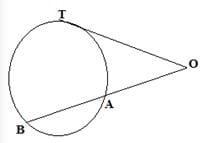Let the tangent be T

OT2 = OA.OB

=> OA = 4

=> OB = OA + AB

=> 4 + 60

=> 64

OT2 = 4 x 64

=> 256

=> OT = 16 cm

RRB JE ME (CBT I) Mock Test- 1 - Question 18

A copper wire, when bent in the form of a square, enclose an area of 121 cm2. If the same wire is bent in the form of a circle, then the area of the circle is

Detailed Solution for RRB JE ME (CBT I) Mock Test- 1 - Question 18 Side of square = √121 = 11 cm

Length of wire = circumference of circle.

44 = 2 πr or r = (44 x 7)/(2 x 22) = 7 cm

Hence, area of circle = πr2 = 22/7 x 7 x 7 = 154 cm2

RRB JE ME (CBT I) Mock Test- 1 - Question 19

Table given below shows the total number of employees of four different grades in a department and the average age of employees in those different grades.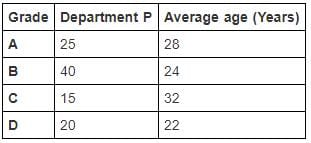The table given below shows the total number of employees of four different grades in a department and the average age of employees in those different grades.

Detailed Solution for RRB JE ME (CBT I) Mock Test- 1 - Question 19 Sum of ages of all employees in grade A = 25 x 28 = 700 years

Sum of ages of all employees in grade B = 40 x 24 = 960 years

Sum of ages of all employees in grade C = 15 x 32 = 480 years

Sum of ages of all employees in grade D = 20 x 22 = 440 years

Sum of ages of all the employees of the department P = 700 + 960 + 480 + 440 = 2580 years

Total employees in department P = 25 + 40 + 15 + 20 = 100

Average age of the employees of department P = 2580/100 = 25.8 years

RRB JE ME (CBT I) Mock Test- 1 - Question 20

What will be the percentage profit after selling an article at a certain price if there is a loss of 12.5% when the article is sold at half of the previous selling price?

Detailed Solution for RRB JE ME (CBT I) Mock Test- 1 - Question 20 Suppose the previous selling price = Rs. x

Selling price = Rs. x/2

There is a loss of 12.5% when the selling price is Rs. x/2.

Cost Price = x/2 x 100/(100 - 12.5) = (100 x)/175 = 4x/7

Now, when selling price is Rs. x, % profit = [(x - (4x/7))/(4x/7)] x 100 = (3x/4x) x 100 = 75%

RRB JE ME (CBT I) Mock Test- 1 - Question 21

If α, 3α+6, and 71α are in arithmetic progression, then find the value of α.

Detailed Solution for RRB JE ME (CBT I) Mock Test- 1 - Question 21 α, 3α + 6, and 71α are in arithmetic progression.

=> 3α + 6 - α = 71α - 3α - 6 = common difference of the arithmetic progression.

=> 2α = 68α - 6 - 6

=> 66α = 12

=> α = 12/66 = 2/11

RRB JE ME (CBT I) Mock Test- 1 - Question 22

One-fourth of Lainey's savings in Kisan Vikas Patra is equal to one-fifth of her savings in the Employee Provident Fund. How much has she saved in Employee Provident Fund if she has a total savings of ₹ 180,000?

Detailed Solution for RRB JE ME (CBT I) Mock Test- 1 - Question 22 Let her savings in Employee Provident Fund be ₹ x.

∴ Her savings in Kisan Vikas Patra = ₹ (180,000 - x)

∴ (180,000 - x)/4 = x/5

=> 900,000 - 5x = 4x

=> x = ₹ 100,000

RRB JE ME (CBT I) Mock Test- 1 - Question 23

When 20% of 40% of half of a number 'm' is added to 3/4th of 20% of itself, then a second number is obtained, which is 21 less than the 40% of m. What is the difference between the first and second number?

Detailed Solution for RRB JE ME (CBT I) Mock Test- 1 - Question 23 First number = m

Second number = (40% of m ) - 21

According to the question:

20% of 40% of (m/2) + (3/4) x 20% of m = (40% of m) - 21

m = 100

Second number = (40% of m) - 21 = 40% of 100 - 21 = 19

Difference = 100 - 19 = 81

RRB JE ME (CBT I) Mock Test- 1 - Question 24

What will come in place of (?) in this equation?

125% of [{? + 200 ÷ (16 ÷ 2 x 4 x (1/8))} ÷ 7.5 x 8 ÷ (18 ÷ 3 - 50% of 4)] = ?

Detailed Solution for RRB JE ME (CBT I) Mock Test- 1 - Question 24 Let '?' be denoted by 'a'.

125% of [{a + 200 ÷ (16 ÷ 2 x 4 x (1/8))} ÷ 7.5 x 8 ÷ (18 ÷ 3 - 50% of 4)] = a

=> 125% of [{a + 200 ÷ (8 x 4 x (1/8))} ÷ 7.5 x 8 ÷ (6 - 2)] = a

=> 125% of [{a + 200 ÷ 4} ÷ 7.5 x 8 ÷ 4] = a

=> 125% of [{a + 50} ÷ 7.5 x 2] = a => 125% of 2(a + 50)/7.5 = a

=> (5/4) x 2(a + 50)/7.5 = a

=> a + 50 = 3a

=> a = 25

RRB JE ME (CBT I) Mock Test- 1 - Question 25

If cos (900 - θ)/cos (300 + θ) = 1, then the value of sin θ + cos 2θ

Detailed Solution for RRB JE ME (CBT I) Mock Test- 1 - Question 25 cos (900 - θ)/cos (300 + θ) = 1

sin θ = cos (300 + θ)

sin θ = sin (900 - 300 - θ)

θ = 900 - 300 - θ

θ = 300

= (sin θ + cos 2θ)

= sin 300 + cos 600

= 1/2 + 1/2

= 1

RRB JE ME (CBT I) Mock Test- 1 - Question 26

A fraction is such that if it is squared and then the numerator is divided by 2, while the denominator is increased by 25%, the new fraction thus obtained is 4 times the original fraction. What is the new fraction?

Detailed Solution for RRB JE ME (CBT I) Mock Test- 1 - Question 26 Let the original fraction be x/y

According to the given condition,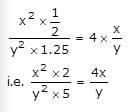RRB JE ME (CBT I) Mock Test- 1 - Question 27

What should come in place of question mark (?) in the following series?

15, 17, 23, 35, 55, (?)

Detailed Solution for RRB JE ME (CBT I) Mock Test- 1 - Question 27 15 + (1 x 2) = 17

17 + (2 x 3) = 23

23 + (3 x 4) = 35

35 + (4 x 5) = 55

55 + (5 x 6) = 85

RRB JE ME (CBT I) Mock Test- 1 - Question 28

How much does a watch lose per day if its hands coincide every 65 minutes?

Detailed Solution for RRB JE ME (CBT I) Mock Test- 1 - Question 28 For a regular clock, we know that the hands of a watch meet after every 65(5/11) minutes.

Hence loss in 65 minutes = 65(5/11) - 65 = (5/11) minutes

Hence loss in 24 hours = (5 x 24 x 60) / (11 x 65) = 10(10/143) minutes

RRB JE ME (CBT I) Mock Test- 1 - Question 29

After fifteen years, the ratio of the ages of a man and his son will be 2: 1. If the present age of the man is 45, then what is his son's present age?

Detailed Solution for RRB JE ME (CBT I) Mock Test- 1 - Question 29 After fifteen years, the ratio of age = 2: 1.

Let the present age of the son be 'x'.

(45 + 15)/(x + 15) = 2/1

2x + 30 = 60 x = 15

So, the present age of son is 15 years

RRB JE ME (CBT I) Mock Test- 1 - Question 30

Ramesh can do 20% more work as Mohan can do at the same time. If Mohan can finish the work in 30 hours, then Ramesh, with the help of Mohan, can finish the same work in how many hours?

Detailed Solution for RRB JE ME (CBT I) Mock Test- 1 - Question 30 Mohan can complete work in 30 hours.

Ramesh can do 6/5 work in 30 hours.

So Ramesh can complete the work in 30 x (5/6) = 25 hours.

Together they can finish 1/30 + 1/25 of work in 1 hour, i.e., 11/150.

So in 150/11 hours, they will finish the work together.

RRB JE ME (CBT I) Mock Test- 1 - Question 31

In the following question, select the related word from the given alternatives.

PARTS: STRAP:: WOLF:?

Detailed Solution for RRB JE ME (CBT I) Mock Test- 1 - Question 31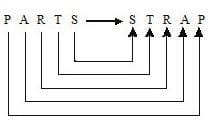similarly,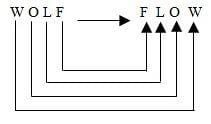RRB JE ME (CBT I) Mock Test- 1 - Question 32

DIRECTIONS: In the question below, there are few statements followed by few conclusions. You have to take the given statements to be true even if they seem to be at variance with commonly known facts and then decide which of the given conclusion logically follow(s) from the given statements.

Statements: All books are pins.

Some pins are desks.

Conclusions: I. Some desks are books.

II. All desks are pins.

III. No desk is book.

Detailed Solution for RRB JE ME (CBT I) Mock Test- 1 - Question 32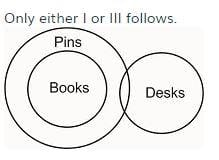RRB JE ME (CBT I) Mock Test- 1 - Question 33

DIRECTIONS: Read the following information carefully and answer the questions given below it.

‘X + Y’ means ‘X is the father of Y’.

‘X – Y’ means ‘X is the mother of Y.’

‘X x Y’ means ‘X is the brother of Y.’

‘X ÷ Y’ means ‘X is the sister of Y’.

Which of the following symbols should replace the question mark in the given expression in order to make the relation ‘P is the niece of M’ definitely true?

M x N ? R – P÷S

Detailed Solution for RRB JE ME (CBT I) Mock Test- 1 - Question 33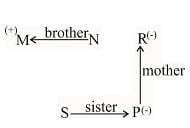M and R must be siblings. So, their intermediary sibling N should be either a brother or a sister of both. Therefore, either x or ÷ can be placed.

RRB JE ME (CBT I) Mock Test- 1 - Question 34

DIRECTIONS: In the question below, there are few statements followed by few conclusions. You have to take the given statements to be true even if they seem to be at variance with commonly known facts and then decide which of the given conclusion logically follow(s) from the given statements.

Statements: All books are pins.

Some pins are desks.

Conclusions: I. Some desks are books.

II. All desks are pins.

III. No desk is book.

Detailed Solution for RRB JE ME (CBT I) Mock Test- 1 - Question 34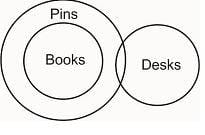RRB JE ME (CBT I) Mock Test- 1 - Question 35

DIRECTIONS: In the questions given below, some relationship has been expressed through symbols as shown below. Based on the meaning of these symbols and choose the correct answer.

ϕ means ‘less than.’

Δ means ‘not greater than.’

- means ‘equal to.’

+ means ‘not equal to.’

× means ‘not less than.’

= means ‘greater than.’

X ϕ Y + Z implies

Detailed Solution for RRB JE ME (CBT I) Mock Test- 1 - Question 35 Clearly, the meaning of the given symbols

X ϕ Y + Z ⇒ X < Y ≠ Z

Using the proper notations/symbols in option (c), we get

X △ Y ϕ Z

⇒ X< Y< Z.

⇒ X< Y ≠ Z.

Therefore, X ϕ Y + Z = X △ Y < ϕ Z.

RRB JE ME (CBT I) Mock Test- 1 - Question 36

DIRECTIONS: In the following question, continuous pattern series is given. Some of the letters of the series are missing. These missing letters are given in that order as one of the four alternatives below the series. Find out the correct alternatives.

jk_ljkk_ljj_klljk_ljk_ll

Detailed Solution for RRB JE ME (CBT I) Mock Test- 1 - Question 36 Series pattern is:

jkkl/jkkll/jjkkll/jkkl/jkkll

∴ Required answer = klkkk

RRB JE ME (CBT I) Mock Test- 1 - Question 37

Roy walks 2 km to the East, then turns North-West and walks 3 km. Then he turns South and walks 5 km. Then again, he turns West and walks 2 km. Finally, he turns North and walks 6 km. In which direction is he from the starting point?

Detailed Solution for RRB JE ME (CBT I) Mock Test- 1 - Question 37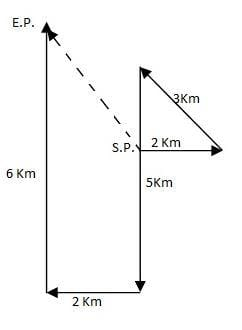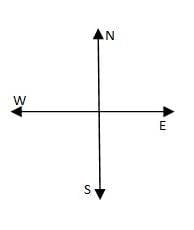Now Roy is in the North-west direction from starting point.

RRB JE ME (CBT I) Mock Test- 1 - Question 38

If’ A’ is coded as 1, ‘B’ as 3, ‘C’ as 5 and so on, which of the following is the numerical value of the word ‘FAZED’?

Detailed Solution for RRB JE ME (CBT I) Mock Test- 1 - Question 38 Given that;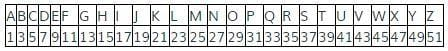So from the above table, numerical value of FAZED= 11 +1 + 51 + 9 + 7 =79.

RRB JE ME (CBT I) Mock Test- 1 - Question 39

If ‘MATCH’ is coded as ‘NCWGM’ and ‘BOX’ as ‘CQA’, then which of the following is coded as ‘OQWIGUVS’?

Detailed Solution for RRB JE ME (CBT I) Mock Test- 1 - Question 39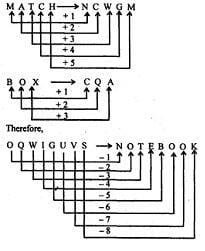RRB JE ME (CBT I) Mock Test- 1 - Question 40

In the question, which one of the given choices would be a meaningful order of the following words?

1. Bibliography

2. Contents

3. Title

4. Chapters

5. Introduction

Detailed Solution for RRB JE ME (CBT I) Mock Test- 1 - Question 40 3. The title "Because it is the first thing a person wants to know about a book".

2. Contents "Because it shows what is in the book".

5. Introduction "Because it introduces the book".

4. Chapters "Because after the introduction we go on to read the chapters".

1. Bibliography "Because this lists the references of the content at the end."

RRB JE ME (CBT I) Mock Test- 1 - Question 41

A word is represented by only one sequence of numbers as given in any one of the alternatives. The sets of numbers given in the alternatives are represented by two classes of alphabets as in the two matrices given below. The columns and rows of Matrix – I are numbered from 0 to 3, and that Matrix – II are numbered from 4 to 7. A letter from these matrices can be represented first by its row and next by its column. e.g., ‘A’ can be represented by 00, 12 etc., and ‘L’ can be represented by 46, 54, 67 etc. Similarly, You have to identify the set for the word given below. “TUBULE”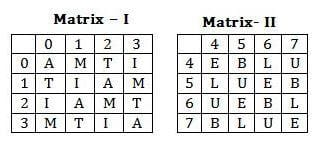Detailed Solution for RRB JE ME (CBT I) Mock Test- 1 - Question 41 TUBULE

Possible code for T: 02/10/23/31

Possible code for U: 47/55/64/76

Possible code for B: 45/57/66/74

Possible code for L: 46/54/67/75

Possible code for E: 44/56/65/77

Hence, the code for TUBULE = 31, 76, 57, 47, 67, 56

RRB JE ME (CBT I) Mock Test- 1 - Question 42

DIRECTIONS: Choose the missing terms out of the given alternatives.

QPO, SRQ UTS, WVU,?

Detailed Solution for RRB JE ME (CBT I) Mock Test- 1 - Question 42 The given sequence of a group of letters follows the pattern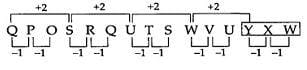Therefore, the missing group of letters = YXW.

RRB JE ME (CBT I) Mock Test- 1 - Question 43

Find out missing number:

9 : 81 :: 12 : ?

Detailed Solution for RRB JE ME (CBT I) Mock Test- 1 - Question 43 9 : (9)2 :: 12 : (12)

= 9 : 81 :: 12 : 144.

RRB JE ME (CBT I) Mock Test- 1 - Question 44

DIRECTIONS: A piece of paper is opened after being cut, as shown below in the questions figure. From the given answer figures, indicate how it will appear when closed?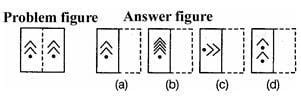Detailed Solution for RRB JE ME (CBT I) Mock Test- 1 - Question 44 When we will open the problem figure, it will look like answer fig. (a)
RRB JE ME (CBT I) Mock Test- 1 - Question 45

DIRECTIONS: Choose the missing terms out of the given alternatives.

B M f q J U ? ?.

Detailed Solution for RRB JE ME (CBT I) Mock Test- 1 - Question 45 The given sequence is a combination of a small-cap letter that follows the pattern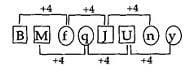Therefore, missing letters =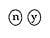RRB JE ME (CBT I) Mock Test- 1 - Question 46

DIRECTIONS: Find out missing number from the following given figures.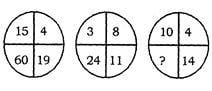Detailed Solution for RRB JE ME (CBT I) Mock Test- 1 - Question 46 In figure I = 15 × 4 = 60,

15 + 4 = 19

In figure II = 3 x 8 = 24,

3 + 8 = 11

Then missing number is = 10 × 4

= 40

RRB JE ME (CBT I) Mock Test- 1 - Question 47

The given alternative choose the one that is different from the other three alternatives.

Detailed Solution for RRB JE ME (CBT I) Mock Test- 1 - Question 47 All other terms except snobbery are related to crimes.
RRB JE ME (CBT I) Mock Test- 1 - Question 48

DIRECTIONS: Select from answer choices a number to replace the question mark.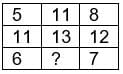Detailed Solution for RRB JE ME (CBT I) Mock Test- 1 - Question 48 (right term × 2) – left term = middle term

(8 × 2) – 5 = 11

(12 × 2) – 11 = 13

So,

(7 × 2) – 6 = 8

RRB JE ME (CBT I) Mock Test- 1 - Question 49

Choose the correct water image of the figure from responses given below?

Question Figure: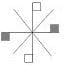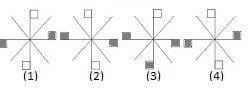Detailed Solution for RRB JE ME (CBT I) Mock Test- 1 - Question 49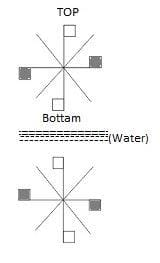RRB JE ME (CBT I) Mock Test- 1 - Question 50

A, B, C, D, E, F and G are members of a family consisting of 4 adults and 3 children, two of whom, F and G, are girls. A and D are brothers, and A is a doctor. E is an engineer married to one of the brothers and has two children. B is married to D, and G is their child. Who is C?

Detailed Solution for RRB JE ME (CBT I) Mock Test- 1 - Question 50 ‘↔’ → brothers, ‘=’ → couple, ‘↓’ → offspring, ‘¨’ → male, ‘O’ → female, ‘X’ → unknown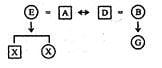Clearly, C and F are the remaining members to be adjusted in place of two x. since there are 3 children out of which two are girls, i.e. G and F, so clearly the third children C, is a boy. So C is the son of E and A.

RRB JE ME (CBT I) Mock Test- 1 - Question 51

DIRECTIONS: Study the following diagram and answer the questions that follow.

a. The rectangle represents men.

b. Circle represents graduates.

c. Triangle represents skilled persons.

d. Square represents employed persons.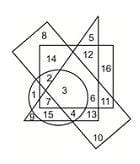The skilled employed men who are not graduates are-

Detailed Solution for RRB JE ME (CBT I) Mock Test- 1 - Question 51 only 6
RRB JE ME (CBT I) Mock Test- 1 - Question 52

Three of the following four are alike in a certain way, and so form a group. Which is the one that does not belong to that group?

Detailed Solution for RRB JE ME (CBT I) Mock Test- 1 - Question 52 Except for Ayurveda, all others are Vedas. ‘Ayurveda’ is the branch of medicine.
RRB JE ME (CBT I) Mock Test- 1 - Question 53

DIRECTIONS: Arrange the following words according to the English dictionary.

(a) Assistant

(b) Assessment

(c) Asbestos

(d) Asterisk

(e) Ass

Detailed Solution for RRB JE ME (CBT I) Mock Test- 1 - Question 53 The correct arrangement is:

(c) Asbestos

(e) Ass

(b) Assessment

(a) Assistant

(d) Asterisk

RRB JE ME (CBT I) Mock Test- 1 - Question 54

Kannan walked 10 km towards North. From there, he turned back and walked 6 km towards South. Then he walked 3 km towards East. How far was he from the starting point?

Detailed Solution for RRB JE ME (CBT I) Mock Test- 1 - Question 54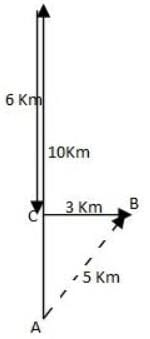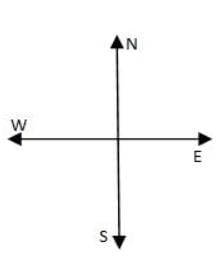From the figure, its clear that Kannan is 5 Km from the starting point.

RRB JE ME (CBT I) Mock Test- 1 - Question 55

DIRECTIONS: Choose the odd one out from the given alternatives.

Detailed Solution for RRB JE ME (CBT I) Mock Test- 1 - Question 55 Except LAMIA, all other groups of letters, if arranged from study subject, i.e. HINDI, SCIENCE, and GEOGRAPHY respectively.
RRB JE ME (CBT I) Mock Test- 1 - Question 56

2023 ICC Cricket World Cup will be hosted completely in which of the following countries?

Detailed Solution for RRB JE ME (CBT I) Mock Test- 1 - Question 56 The 2023 Cricket World Cup will be the 13th edition of the Cricket World Cup, scheduled to be hosted by India, from 9 February to 26 March 2023. This will be the first time the competition is held completely in India.
RRB JE ME (CBT I) Mock Test- 1 - Question 57

The 33rd ASEAN Summit is being chaired by whom?

Detailed Solution for RRB JE ME (CBT I) Mock Test- 1 - Question 57 The summit is being chaired by Singapore’s Prime Minister Lee Hsien Loong.
RRB JE ME (CBT I) Mock Test- 1 - Question 58

In each of the following questions, select a figure from amongst the four alternatives, which, when placed in the blank space of figure (X), would complete the pattern.

Identify the figure that completes the pattern.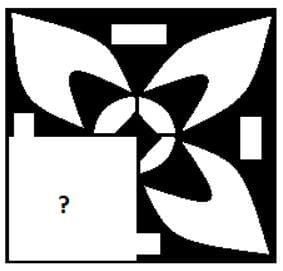Detailed Solution for RRB JE ME (CBT I) Mock Test- 1 - Question 58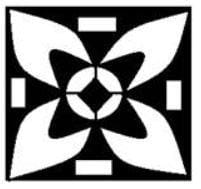RRB JE ME (CBT I) Mock Test- 1 - Question 59

Tughlaqabad, a ruined fort situated in Delhi, was built by Ghiyas-ud-din Tughlaq, the founder of the Tughlaq dynasty of the Delhi Sultanate.

Detailed Solution for RRB JE ME (CBT I) Mock Test- 1 - Question 59 Article 110 of the Constitution defines the “Money Bill.”
RRB JE ME (CBT I) Mock Test- 1 - Question 60

Wrestler Vinesh Phogat has become the first Indian women athlete to win the gold medal at the Asian Games 2018 held in Jakarta, Indonesia. She hails from:

Detailed Solution for RRB JE ME (CBT I) Mock Test- 1 - Question 60 Wrestler Vinesh Phogat has become the first Indian women athlete to win the gold medal at the Asian Games 2018 held in Jakarta, Indonesia. She hails from Haryana.
RRB JE ME (CBT I) Mock Test- 1 - Question 61

India-Korea business summit 2018 was held in:

Detailed Solution for RRB JE ME (CBT I) Mock Test- 1 - Question 61 India-Korea business summit 2018 was held in New Delhi.
RRB JE ME (CBT I) Mock Test- 1 - Question 62

The upcoming General Elections to be held in 2019 will lead to the formation of the _________ Lok Sabha for the Republic of India.

Detailed Solution for RRB JE ME (CBT I) Mock Test- 1 - Question 62 The current Lok Sabha is the 16th since the formation of the Indian Republic.

The General elections of 2019 will elect members for the formation of the 17th Lok Sabha.

RRB JE ME (CBT I) Mock Test- 1 - Question 63

Headquarters of the Indian Space Research Organisation is in _________________

Detailed Solution for RRB JE ME (CBT I) Mock Test- 1 - Question 63
• The Indian Space Research Organisation is the space agency of the Government of India headquartered in Bengaluru.

• It was founded in 1969 by Dr Vikram Sarabhai.

• Its current Chairman is K Sivan.

RRB JE ME (CBT I) Mock Test- 1 - Question 64

"Hampi ruins" is the Heritage architecturally important monumental site in India which is situated in which Indian state?

Detailed Solution for RRB JE ME (CBT I) Mock Test- 1 - Question 64
• Hampi monuments are a subset of the wider-spread Vijayanagar ruins situated in the Indian state of Karnataka.

• It is designated as a UNESCO world heritage site.

RRB JE ME (CBT I) Mock Test- 1 - Question 65

________________ is a security force of India entrusted with protecting railway passengers, passenger area and railway property of the Indian Railways

Detailed Solution for RRB JE ME (CBT I) Mock Test- 1 - Question 65
• Under the Authority of the Ministry of Railways, Railway Police Force (RPF) has the power to arrest, investigate and prosecute criminals.

• Its duty is towards protecting railway passengers, passenger area and railway property of the Indian Railways.

RRB JE ME (CBT I) Mock Test- 1 - Question 66

Government of India launched ____________ mobile App for Freight Managers which provides features for monitoring and managing freight business using Geographic Information System (GIS) Views and Dashboard.

Detailed Solution for RRB JE ME (CBT I) Mock Test- 1 - Question 66 With the use of the SFOORTI application, the movement of freight trains on the Geographic Information System (GIS) view can be tracked. Both passenger and freight trains can be tracked over Zones/Divisions. Freight Business will get a boost due to the application monitoring.
RRB JE ME (CBT I) Mock Test- 1 - Question 67

Ranji trophy is associated with which sport?

Detailed Solution for RRB JE ME (CBT I) Mock Test- 1 - Question 67
• The Ranji Trophy is a domestic first-class cricket championship played in India between teams representing regional and state cricket associations.

• The competition currently consists of 37 teams, with all 29 states in India and two of the seven union territories having at least one representation.

RRB JE ME (CBT I) Mock Test- 1 - Question 68

2 + 2 Ministerial Dialogue is a Bilateral diplomatic conference between India and __________

Detailed Solution for RRB JE ME (CBT I) Mock Test- 1 - Question 68
• 2 + 2 Ministerial Dialogue is a Bilateral Annual conference between the USA and India.

• It was conceptualised in 2017 following the Official visit of PM Modi to the United States.

• The dialogue pans a wide range of Strategic issues, including Defence, Economy, Trade etc.

RRB JE ME (CBT I) Mock Test- 1 - Question 69

The first railway on Indian sub-continent ran over a stretch of 21 miles from __________ to _____________ in the 19th century

Detailed Solution for RRB JE ME (CBT I) Mock Test- 1 - Question 69
• Asia's first railway train chugged out from Bori Bunder, Bombay and reached faraway Tannah (today's Thane) on April 16, 1853.

• Headquartered in New Delhi, today, the Indian Railway manages the fourth-largest railway network in the world by size, with 121,407 kilometres of total track over a 67,368-kilometre route.

RRB JE ME (CBT I) Mock Test- 1 - Question 70

Indian economy is expected to grow at ______ in 2018-19 according to the Central Statistics Office.

Detailed Solution for RRB JE ME (CBT I) Mock Test- 1 - Question 70 Releasing the first advance estimates of National Income for 2018-19, the CSO said, "The growth in GDP during 2018-19 is estimated at 7.2% as compared to the growth rate of 6.7% in 2017-18."
RRB JE ME (CBT I) Mock Test- 1 - Question 71

Time period of a pendulum depends on which of the following?

Detailed Solution for RRB JE ME (CBT I) Mock Test- 1 - Question 71 The time period of a pendulum depends on the length of the pendulum & acceleration due to gravity.

The time period of a pendulum is directly proportional to the length of the pendulum and inversely proportional to the acceleration due to gravity.

• if the length is increases, time period increases and vice-versa &

• If acceleration due to gravity is increased, the time period decreased and vice-versa

RRB JE ME (CBT I) Mock Test- 1 - Question 72

Which of the following are the ores from which iron is extracted industrially?

Detailed Solution for RRB JE ME (CBT I) Mock Test- 1 - Question 72
• Haematite and Magnetite are the chief ores of iron extraction industrially. Haematite: Fe2O3; Magnetite: Fe3O4

• Iron ore is the main ingredient used in Steel producing industries.

• India is the 4th largest iron ore producing country in the world. The states where iron ores are chiefly extracted for industrial purposes are- Orissa, Karnataka, Chhattisgarh, Goa and Jharkhand.

RRB JE ME (CBT I) Mock Test- 1 - Question 73

Find the distance travelled by a body moving with an initial velocity of 15 m/s and constant acceleration of 3 m/s2 in the time period of 15 seconds from the point of the initial velocity.

Detailed Solution for RRB JE ME (CBT I) Mock Test- 1 - Question 73 Using the distance-time equation for a body moving with constant acceleration,

S = ut +1/2at2 where, S = distance travelled, u = initial velocity, a = acceleration, t = time taken for body to travel distance s.

u = 0 m/s, a = 3 m/s2, t = 15 s;

Putting the values in the equation, S = ut +1/2at2

S = 4 x 15 + ½ (3 x 15 x 15)

or S = 60 + 337.50

or S = 397.50 m

RRB JE ME (CBT I) Mock Test- 1 - Question 74

____________ is the study of animals and their characteristics while ____________ is the study of plants and its nature.

Detailed Solution for RRB JE ME (CBT I) Mock Test- 1 - Question 74
• Zoology is the scientific study of animals and their characteristics, while Botany is studying plants and their nature.

• Botany, along with the study of plants,, also included the study of fungi and algae.

• Botanical research has various applications in food and textiles industries, in modern horticulture, agriculture and forestry, plant propagation, breeding, and genetic modification.

• Zoological studies deal with the structure, embryology, evolution, classification, habits, and distribution of all animals, both living and extinct.

RRB JE ME (CBT I) Mock Test- 1 - Question 75

Which of the following involves delivering powerful waves of energy to disrupt the ability of cancer cells to grow and divide?

Detailed Solution for RRB JE ME (CBT I) Mock Test- 1 - Question 75
• Radiation therapy involves powerful waves of energy delivered in small doses to disrupt the ability of cancer cells to grow and divide, killing cancer cells or even slowing their growth and shrinking tumours to enable surgery on the affected part.

• Radiation Therapy is performed to have a targeted, powerful effect on tumours confined to a specific area in the body.

RRB JE ME (CBT I) Mock Test- 1 - Question 76

Which of the following are TRUE regarding the element, Tungsten?

Detailed Solution for RRB JE ME (CBT I) Mock Test- 1 - Question 76 Following are the properties of Tungsten:

Atomic No.- 74, Periodic Table group- 6

• Tungsten is used for the filaments of incandescent light bulbs

• It has the highest melting point of all metals

• The melting point is 3414°C

• It is a shiny, silvery-white metal extracted from its chief ores, scheelite and wolframite.

RRB JE ME (CBT I) Mock Test- 1 - Question 77

What is the Chemical formulae of Benzene?

Detailed Solution for RRB JE ME (CBT I) Mock Test- 1 - Question 77
• The Chemical Formulae of Benzene is C6H6. It is a colourless, flammable, aromatic hydrocarbon.

• Benzene has a ring structure where all the carbon-carbon bonds in the molecule are of the same length.

• Benzene is used in the manufacturing of products like plastics, resins, synthetic fibres, rubber lubricants, dyes, detergent.

RRB JE ME (CBT I) Mock Test- 1 - Question 78

In an electrical circuit, If Voltage is kept constant and Resistance is increased, the current in the circuit will_____________.

Detailed Solution for RRB JE ME (CBT I) Mock Test- 1 - Question 78 Expression of Ohm's Law is given by: V = I R

Where, V = voltage, I = Current, R = Resistance.

So, if Resistance is increased by keeping the voltage constant, the Current in the circuit will decrease.

RRB JE ME (CBT I) Mock Test- 1 - Question 79

Which of the following is/are TRUE regarding Sound waves?

Detailed Solution for RRB JE ME (CBT I) Mock Test- 1 - Question 79
• Sound waves are longitudinal in nature in the air or any fluid medium in which it travels.

• Sound waves are mechanical waves, so it needs medium to travel.

• A mechanical wave propagates as an oscillation of matter (medium) and transfers energy through a medium.

• The sound waves travelling in a medium always vibrate parallel to the direction that the wave moves.

• So, no medium would render sound waves not getting propagated at all as in a vacuum.

RRB JE ME (CBT I) Mock Test- 1 - Question 80

Freezing point of Nitrogen is _______.

Detailed Solution for RRB JE ME (CBT I) Mock Test- 1 - Question 80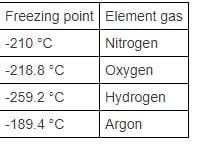RRB JE ME (CBT I) Mock Test- 1 - Question 81

Which among the following is a unit of Force?

Detailed Solution for RRB JE ME (CBT I) Mock Test- 1 - Question 81 Dyne is a unit of force.
• Poise- Dynamic Viscosity

• Barye- Pressure

• Calorie- Heat Energy

RRB JE ME (CBT I) Mock Test- 1 - Question 82

Mojo is suffering from a common eye condition where his vision of nearby objects appears blurred. He is suffering from _____________

Detailed Solution for RRB JE ME (CBT I) Mock Test- 1 - Question 82 Hypermetropia or Long-sightedness is an eye condition where the person's vision of nearby objects is blurred.

The cause of it happening may be genetic.

The condition of Hypermetropia can be corrected by:

• Using glasses of required power

• Using contact lenses for the eye

• By laser Surgery of the eye

RRB JE ME (CBT I) Mock Test- 1 - Question 83

Which of the following phenomenon is responsible for the formation of a soap bubble?

Detailed Solution for RRB JE ME (CBT I) Mock Test- 1 - Question 83
• The surface tension of water provides the necessary wall tension that pulls the bubbles into spherical shapes resulting in the formation of bubbles with water.

• Surface tension is also responsible for the shape acquired by a liquid droplet.

RRB JE ME (CBT I) Mock Test- 1 - Question 84

Sun and other stars produce light energy due to which of the following process?

Detailed Solution for RRB JE ME (CBT I) Mock Test- 1 - Question 84
• Sun and other stars produce light energy due to the Nuclear fusion reactions taking place in them.

• Nuclear fusion is a process where energy is produced by combining two lighter atoms.

• Hydrogen atoms in the Sun or other stars undergo Nuclear fusion to form Helium under extreme temperature and pressure.

RRB JE ME (CBT I) Mock Test- 1 - Question 85

Value of 'g', acceleration due to gravity acting on an object increases when_________.

Detailed Solution for RRB JE ME (CBT I) Mock Test- 1 - Question 85
• Acceleration due to gravity increases when there is a decrease in altitude. We know that, More nearer the objects greater the gravitational force.

• So, the gravitational force is inversely proportional to the distance between the two objects.

• Therefore, as the altitude decrease, the distance of the object from the earth's centre also decreases, resulting in an increase in ‘g.’

• Since the mass of any object is always going to be very small compared to the mass of the earth, that factor is considered negligible.

RRB JE ME (CBT I) Mock Test- 1 - Question 86

Which of the following carries the fresh oxygen-rich blood pumped in the heart to different tissues of the body?

Detailed Solution for RRB JE ME (CBT I) Mock Test- 1 - Question 86 Arteries are responsible for carrying the fresh oxygen-rich blood pumped in the heart to different body tissues.

Characteristics of Arteries in the human body are:

• carry blood away from the heart to the tissues of the body

• are usually positioned deeper within the body

• are more muscular than veins, which helps in transporting blood that is full of oxygen efficiently to the tissues. Veins, on the other hand, carry blood from the oxygen-depleted tissues of the body back to the heart for purification.

RRB JE ME (CBT I) Mock Test- 1 - Question 87

Which of the following is a precision measuring tool?

Detailed Solution for RRB JE ME (CBT I) Mock Test- 1 - Question 87 Vernier Callipers is a precision measuring tool used in physics experiments.

It can be used to measure:

• Outside distance such as the length of an object

• Inside distance, such as the width of a groove with extreme precision.

It is a small movable scale used for obtaining fractional parts of subdivisions on a fixed main scale of any measuring instrument.

RRB JE ME (CBT I) Mock Test- 1 - Question 88

Neutralization is a type of chemical reaction where, a ___________ & a ____________ react to form a salt and water.

Detailed Solution for RRB JE ME (CBT I) Mock Test- 1 - Question 88 When a Strong Acid and a Strong Base react, they form salt and water as a result. This is called a Neutralization reaction.

When a cation (positive ion) of a base form a compound with the anion (negative ion) of an acid, the salt is formed with a pH value of 7, which is a neutral value.

Example: HCL (Strong Acid) + NaOH (Strong Base) à H2O + NaCl (Salt)

RRB JE ME (CBT I) Mock Test- 1 - Question 89

Which of the following chiefly form the alloy, Bronze?

Detailed Solution for RRB JE ME (CBT I) Mock Test- 1 - Question 89 Chief compositions for Bronze alloy are Copper and Tin.

Tin is used for making the alloy harder. So, if the alloy is to be made harder for specific purposes, then the content of tin addition is more.

Bronze is used in various applications in everyday life like in Coins, Medals, sculptures or construction of a musical instrument since it has a sonorous property.

RRB JE ME (CBT I) Mock Test- 1 - Question 90

It is a practice to leave some gap between railway tracks, as they tend to expand on a _____________.

Detailed Solution for RRB JE ME (CBT I) Mock Test- 1 - Question 90
• All metal objects tend to expand when they get heated due to any factor. In this case, railway tracks expand on a hot sunny day.

• If two railway tracks were to be laid together without any gap between them, the push caused due to expansion against each other might lead to the derailment of tracks and cause accidents.

RRB JE ME (CBT I) Mock Test- 1 - Question 91

Newton's first law of motion gives which of the following concepts in physics?

Detailed Solution for RRB JE ME (CBT I) Mock Test- 1 - Question 91 Inertia is the property of the matter/body by the virtue of which it resists change of its natural state of movement or rest when the force of change acts.

Newton's first law of mechanics in physics states that:

• An object at rest tends to stay at rest

• An object in motion tends to stay in motion.

RRB JE ME (CBT I) Mock Test- 1 - Question 92

Duodenum is part of __________ in the human system.

Detailed Solution for RRB JE ME (CBT I) Mock Test- 1 - Question 92 Duodenum is the 1st part of the small intestine in the human digestive system where the first phase of digestion is completed.

In this part, the food from the stomach is mixed with enzymes from the pancreas and bile from the gallbladder, which breaks down the food to its simplest form for nutrient absorption.

Other parts of Small Intestine are - Ileum and Jejunum.

RRB JE ME (CBT I) Mock Test- 1 - Question 93

Who among the following is known as The Father of Homeopathy?

Detailed Solution for RRB JE ME (CBT I) Mock Test- 1 - Question 93
• Samuel Hahnemann is regarded as the founder of Homoeopathy for establishing the fundamental principles of the medicinal cure through Homoeopathy.

• He has pioneered the way to prepare medicines in a specialised way and prove them on healthy human beings to determine how the medicines acted to cure diseases.

• He famously espoused the law of cure known as "Similia Similibus Curentur", or "Like Cures Like".

RRB JE ME (CBT I) Mock Test- 1 - Question 94

Determine the resistance value of an appliance operating at 240V and consuming power of 1200 Watts.

Detailed Solution for RRB JE ME (CBT I) Mock Test- 1 - Question 94 Power equation: P= V2/ R; P- Power consumption, V= operating voltage, R- Resistance

According to the question,

P= V2/ R or 1200 = (240 x 240)/ R

or R= 57600/ 1200

or R= 48 ohms

RRB JE ME (CBT I) Mock Test- 1 - Question 95

Mass of a neutron is _______________.

Detailed Solution for RRB JE ME (CBT I) Mock Test- 1 - Question 95 According to the Committee on Data for Science and Technology, the mass of a neutron is 1.6 x 10-27 kg

Mass of proton: Mass of a proton is 1.6726 x 10-27 kg.

Electron's mass is 9.1 x 10-31 kg.

RRB JE ME (CBT I) Mock Test- 1 - Question 96

____________ is a technique using sound waves which are used to map or locate objects in the surrounding environment.

Detailed Solution for RRB JE ME (CBT I) Mock Test- 1 - Question 96 Sound Navigation and Ranging (SONAR) is a technique that uses sound waves to map or locate objects in the surrounding environment.

Working: Firstly, a cluster of sound waves are emitted in the direction of the object. Some of the waves are bounced off, but the majority are reflected back and received by the receiver. This helps in locating objects.

This technique is already used by some species such as bats, whales, dolphins etc., in nature.

RRB JE ME (CBT I) Mock Test- 1 - Question 97

If Blossom has a deficiency of Vitamin C in her body, which of the following disease she might suffer from?

Detailed Solution for RRB JE ME (CBT I) Mock Test- 1 - Question 97 Scurvy is a medical condition that occurs in vitamin C deficiency in the diet.

The human body cannot synthesise vitamin C on its own and depends on external dietary sources to meet its vitamin C needs.

Some of the sources for Vitamin C are: tomatoes, leafy green vegetables like Spinach, cabbage etc.

RRB JE ME (CBT I) Mock Test- 1 - Question 98

Which of the following acid is present in the stomach of the human digestive system?

Detailed Solution for RRB JE ME (CBT I) Mock Test- 1 - Question 98
• Hydrochloric Acid (HCl) is present in the stomach of the human digestive system.

• Hydrochloric acid helps convert pepsinogen into an enzyme called pepsin, which is essential for protein digestion in the the human body.

• Pepsin breaks up the long, folded chains of amino acids into shorter and simpler structures for digestion and absorption in the human system.

RRB JE ME (CBT I) Mock Test- 1 - Question 99

Which of the following acid is present in beverages like Coca-Cola or Appy fizz?

Detailed Solution for RRB JE ME (CBT I) Mock Test- 1 - Question 99 Carbonated drinks like coca-cola or others contain H2CO3

The carbon dioxide present in such beverages forms a very weak carbonic acid (H2CO3) which causes the tingly sensation in the human tongue.

Carbon dioxide is a stable compound with covalent bonds between Carbon and Oxygen atoms.

RRB JE ME (CBT I) Mock Test- 1 - Question 100

Which of the following has a pH value greater than 7?

Detailed Solution for RRB JE ME (CBT I) Mock Test- 1 - Question 100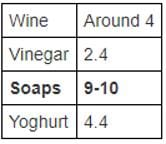## RRB JE Mock Test Series for Mechanical Engineering (ME)

15 tests
 Use Code STAYHOME200 and get INR 200 additional OFF Use Coupon Code
Information about RRB JE ME (CBT I) Mock Test- 1 Page
In this test you can find the Exam questions for RRB JE ME (CBT I) Mock Test- 1 solved & explained in the simplest way possible. Besides giving Questions and answers for RRB JE ME (CBT I) Mock Test- 1, EduRev gives you an ample number of Online tests for practice

15 tests